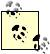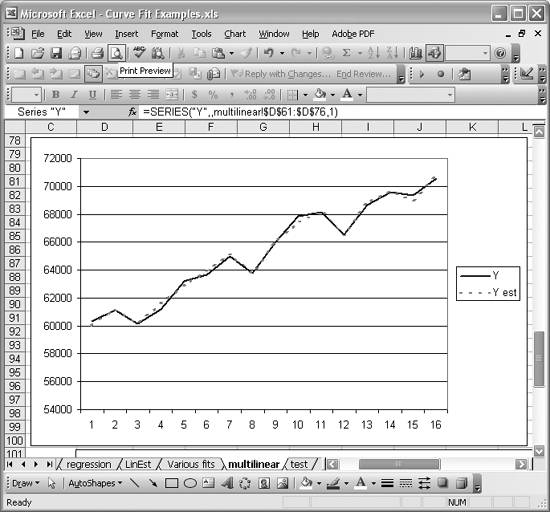# Performing Multiple Linear Regression

Problem

You want to perform multiple linear regression, where an independent variable depends linearly on more than one independent variable.

Solution

Use Excel's LINEST function. LINEST computes statistics for a least-squares straight line through a given set of data. It can be used for linear regression or multiple linear regression. See Recipe 8.3 for an example using LINEST for linear regression. The steps for using LINEST for multiple linear regression are almost identical; the only differences are the model being fit to the data and the number of cells you have to select when entering the array formula.

Discussion

As discussed in Recipe 8.3, the syntax for LINEST is {= LINEST(y-value cell range, x-value cell range, compute intercept, compute statistics)}. Braces surround this formula because it is an array formula. When you type this formula into a cell, you must press Ctrl-Shift-Enter to enter it. Further, you must select a grid of cells before typing and entering the formula. This is because LINEST returns an array of data containing the various statistics computed for the best-fit line. For multiple linear regression, the grid of cells you have to select must be n + 1 columns wide by 5 rows high, where n represents the number of independent variables in the data (the x-variables).

The first argument in LINEST is a cell range containing the y-values for the data to be fit, and the second argument is a cell range containing the x-values, which should be in multiple adjacent columns, corresponding to the number of independent variables in the data being fit. The third argument is a logical value (true or false) specifying whether or not to force the intercept of the fit line to pass through zero. If TRue, the intercept is calculated in the usual least-squares manner. If false, the intercept is forced to zero, with the slope computed accordingly. The fourth argument is a logical value indicating whether or not to display extended statistics for the best-fit line. These extended statistics include such things as standard errors and residual sums.

Let's consider a standard benchmark dataset. I downloaded the data shown in Figure 8-8 from the NIST web site (http://www.nist.gov).[*] The data represents labor statistics where the dependent variable, y, is the Total Derived Employment and the independent variables are GNP Implicit Price Deflator with Year 1954 = 100, Gross National Product, Unemployment, Size of Armed Forces, Non-Institutional Population over Age 14, and Year, corresponding to the variables x1, x2, x3, x4, x5, and x6, respectively.

[*] NIST stands for National Institute of Standards and Technology. The data used here is from the NIST statistical reference library, which is freely available online. The data was originally prepared by J. W. Longley in 1967. See J. W. Longley, "An Appraisal of Least Squares Programs for the Electronic Computer from the Viewpoint of the user." Journal of the American Statistical Association 62 (1967): 819-841.

The model to be fit is of the form y = m6x6 + m5x5 + m4x4 + m3x3 + m2x2 + m1x1 + b. This model has seven parameters (the ms and b) that are determined by the fitting process.

The steps for applying LINEST in this example are as follows:

1. With the mouse, select the cell range D25:J29. (You can select any (n + 1) x 5 range you'd like).
2. Type the LINEST formula using the syntax shown in the formula bar in Figure 8-8.
3. Press Ctrl-Shift-Enter to enter the formula as an array formula.

The results are shown in Figure 8-8. I labeled the model parameters for clarity. Notice the R-square value shown in bold in Figure 8-8. A value of 0.995 represents a very good fit and agrees very well with the results published by the NIST for this dataset.

I also calculated the estimated y-values as shown in column K (Figure 8-8). Figure 8-9 shows a chart of the estimated y-value along with the actual y-value. Visually, the fit very reasonably approximates the underlying data.

Figure 8-8. Multiple linear regression using LinEstOne of the challenges with multiple regression is determining which independent variables best predict the dependent variable without duplicating characteristics. That is, you don't want to use independent variables that are highly correlated with each other. See Recipe 5.4 for more information on conducting correlation analyses in Excel.

Figure 8-9. Y and Y-estimatedExcel Scientific and Engineering Cookbook (Cookbooks (OReilly))
ISBN: 0596008791
EAN: 2147483647
Year: N/A
Pages: 206
Authors: David M Bourg